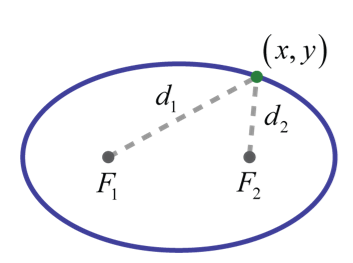# Ellipses in Standard Form Worksheets

What Is Meant By Ellipses in the Standard Form? An ellipse is the set of points in a plane whose distances from two fixed points, called foci, have a sum that is equal to a positive constant. In other words, if points F1F1 and F2F2 are the foci (plural of focus) and dd is some given positive constant then (x, y) (x, y) is a point on the ellipse if d= d1+d2 as pictured below:In addition, an ellipse can be formed by the intersection of a cone with an oblique plane that is not parallel to the side of the cone and does not intersect the base of the cone. Points on this oval shape where the distance between them is at a maximum are called vertices and define the major axis. The center of an ellipse is the midpoint between the vertices. The minor axis is the line segment through the center of an ellipse defined by two points on the ellipse where the distance between them is at a minimum. The endpoints of the minor axis are called co-vertices.

• ### Basic Lesson

Guides students through the beginner skills of using Ellipses in the Standard Form. Write the standard equation of each ellipse.

• ### Intermediate Lesson

Demonstrates how to use advanced skills to tackle writing ellipses in the standard form problems.

• ### Independent Practice 1

A really great activity for allowing students to understand the concepts of the writing ellipses in the standard form.

• ### Independent Practice 2

Students use writing ellipses in the standard form in 20 assorted problems. The answers can be found below.

• ### Homework Worksheet

Students are provided with 12 problems to achieve the concepts of writing ellipses in the standard form.

• ### Skill Quiz

This tests the students ability to understand how to write ellipses in the standard form.

Answers for all lessons and independent practice.